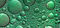Source: Unsplash

# Radial Basis Functions, RBF Kernels, & RBF Networks Explained Simply

## A different learning paradigm

Here is a set of one-dimensional data: your task is to find a way to perfectly separate the data into two classes with one line.

At first glance, this may appear to be an impossible task, but it is only so if we restrict ourselves to one dimension.

Let’s introduce a wavy function f(x) and map each value of x to its corresponding output. Conveniently, this makes all the blue points higher and the red points lower at just the right locations. We can then draw a horizontal line that cleanly divides the classes into two parts.

This solution seems very sneaky, but we can actually generalize it with the help of radial basis functions (RBFs). Although they have many specialized use cases, an RBF inherently is simply a function whose points are defined as distances from a center. Methods that use RBFs fundamentally share a learning paradigm different from the standard machine learning fare, which is what makes them so powerful.

For example, the Bell Curve is an example of a RBF, since points are represented as number of standard deviations from the mean. Formally, we may define an RBF as a function that can be written as:

Note the double pipes (informally, in this use case) represent the idea of ‘distance’, regardless the dimension of x. For example,

• this would be absolute value in one dimension: `f(-3) = f(3)`. The distance to the origin (0) is 3 regardless of the sign.
• this would be Euclidean distance in two dimensions: `f([-3,4]) = f([3,-4])`. The distance to the origin (0, 0) is 5 units regardless of the specific point’s location.

This is the ‘radius’ aspect of the ‘radial basis function’. One can say that radial basis functions are symmetrical around the origin.

The task mentioned above — magically separating points with one line — is known as the radial basis function kernel, with applications in the powerful Support Vector Machine (SVM) algorithm. The purpose of a ‘kernel trick’ is to project the original points into some new dimensionality such that it becomes easier to separate through simple linear methods.

Take a simpler example of the task with three points.

Let’s draw a normal distribution (or another arbitrary RBF function) centered at each of the points.

Then, we can flip all the radial basis functions for data points of one class.

If we add all the values of the radial basis functions at each point x, we an intermediate ‘global’ function that looks something like this:

We’ve attained our wavy global function (let’s call it `g(x)`)! It works with all sorts of data layouts, because of the nature of the RBF function.

Our RBF function of choice — the normal distribution — is dense in one central area and less so in all other places. Hence, it has a lot of sway in deciding the value of g(x) when values of x are near its location, with diminishing power as the distance increases. This property makes RBF functions powerful.

When we map every original point at location `x` to the point `(x, g(x))` in two-dimensional space, the data can always be reliably separated, provided it is not too noisy. It will always be mapped in accordance with proper density of the data because of overlapping RBF functions.

In fact, linear combinations of— adding and multiplying — Radial Basis Functions can be used to approximate almost any function well.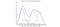A function (black) used to model data points (purple) composed of several RBF functions (solid colorful lines). Source. Image free to share

Radial Basis Networks take this idea to heart by incorporating ‘radial basis neurons’ in a simple two-layer network.

The input vector is the n-dimensional input in which a classification or regression task (only one output neuron) is being performed on. A copy of the input vector is sent to each of the following radial basis neurons.

Each RBF neuron stores a ‘central’ vector — this is simply one unique vector from the training set. The input vector is compared to the central vector, and the difference is plugged into an RBF function. For example, if the central and input vectors were the same, the difference would be zero. The normal distribution at x = 0 is 1, so the output of the neuron would be 1.

Hence, the ‘central’ vector is the vector at the center of RBF function, since it is the input that yields the peak output.

Likewise, if the central and input vectors are different, the output of the neuron decays exponentially towards zero. The RBF neuron, then, can be thought of as a nonlinear measure of similarity between the input and central vectors. Because the neuron is radial — radius-based — the difference vector’s magnitude, not direction, matters.

Lastly, the learnings from the RBF nodes are weighted and summed through a simple connection to the output layer. Output nodes give large weight values to RBF neurons that have specific importance to a category, and smaller weights for neurons whose outputs matter less.

Why does the radial basis network take a ‘similarity’ approach to modelling? Take the following example two-dimensional dataset, where the central vectors of twenty RBF nodes are represented with a ‘+’.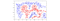Source: McCormick ML. Image free to share.

Then, look at a contour map of the the prediction space for the trained RBF network: around almost every central vector (or group of central vectors) is a peak or a valley. The feature space of the network is ‘defined’ by these vectors, just like how the global function g(x) discussed in RBF kernels is formed by radial basis functions centered at each data point.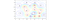Source: McCormick ML. Image free to share.

Because it is impractical to form one RBF node for every single item in the training set like kernels do, radial basis networks chose central vectors to shape the network’s view of the landscape. These central vectors are usually found through some clustering algorithm like K-Means, or alternatively simply through random sampling.

The drawn feature boundary based on height looks like this: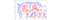Source: McCormick ML. Image free to share.

The radial basis network fundamentally approaches the task of classification differently than standard neural networks because of the usage of a radial basis function, which can be thought of as measuring density. Standard neural networks seek to separate the data through linear manipulations of activation functions, whereas radial basis functions seek more to group the data through fundamentally ‘density’-based transformations.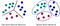Created by author

Because of this, as well as its lightweight architecture and strong nonlinearity, it is a top contender with artificial neural networks.

Fundamentally, applications of radial basis functions rely on a concept called ‘radial basis function interpolation’, which is a topic of great interest in approximation theory, or the study of approximating functions efficiently.

As mentioned previously, RBFs are a mathematical embodiment of the idea that a point should have the most influence at that point and decaying influence for increasing distances from that point. Because of this, they can be manipulated in very simple ways to construct complex nonlinearities.

## Summary / Key Points

• A Radial Basis Function (RBF) is a function that is only defined by distances from a center. Exact position does not matter; only relative position matters.
• Primarily, RBFs are used because of one property: at the center, the output (influence) is highest; at each distance unit away from the center (in any direction) the influence decays.
• RBF kernels place a radial basis function centered at each point, then perform linear manipulations to map points to higher-dimensional spaces that are easier to separate.
• Radial Basis Networks are simple two-layer architectures with one layer of RBF neurons and one layer of output neurons. RBF neurons are each assigned a ‘central vector’, from which input vectors are compared. These networks fundamentally utilize density and hence are able to model complex nonlinearities with very small structures.

Thanks for reading!

All images created by author unless otherwise stated.

## Analytics Vidhya

Analytics Vidhya is a community of Analytics and Data…

## Sign up for Analytics Vidhya News Bytes

### By Analytics Vidhya

Latest news from Analytics Vidhya on our Hackathons and some of our best articles! Take a look.

By signing up, you will create a Medium account if you don’t already have one. Review our Privacy Policy for more information about our privacy practices.

Check your inbox
Medium sent you an email at to complete your subscription.

Written by

## Andre Ye

ML & CS enthusiast. Let’s connect: https://www.linkedin.com/in/andre-ye.

## Analytics Vidhya

Analytics Vidhya is a community of Analytics and Data Science professionals. We are building the next-gen data science ecosystem https://www.analyticsvidhya.com

Written by

## Andre Ye

ML & CS enthusiast. Let’s connect: https://www.linkedin.com/in/andre-ye.## Analytics Vidhya

Analytics Vidhya is a community of Analytics and Data Science professionals. We are building the next-gen data science ecosystem https://www.analyticsvidhya.com

## More From Medium

Medium is an open platform where 170 million readers come to find insightful and dynamic thinking. Here, expert and undiscovered voices alike dive into the heart of any topic and bring new ideas to the surface. Learn more

Follow the writers, publications, and topics that matter to you, and you’ll see them on your homepage and in your inbox. Explore

If you have a story to tell, knowledge to share, or a perspective to offer — welcome home. It’s easy and free to post your thinking on any topic. Write on Medium